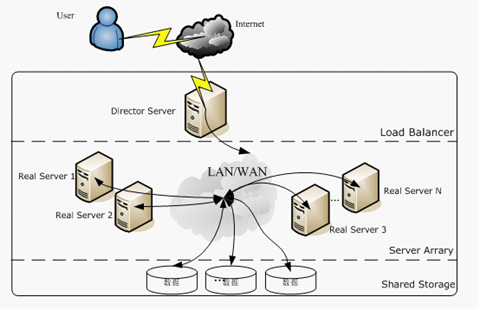## FastText简介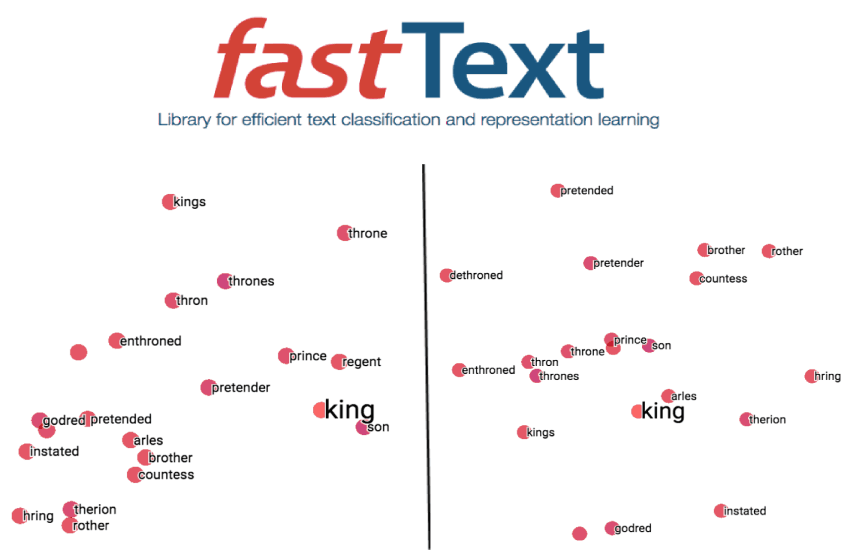• Enriching Word Vectors with Subword Information：这篇论文提出了用 word n-gram 的向量之和来代替简单的词向量的方法，以解决简单 word2vec 无法处理同一词的不同形态的问题。fastText 中提供了 maxn 这个参数来确定 word n-gram 的 n 的大小。
• Bag of Tricks for Efficient Text Classification：这篇论文提出了 fastText 算法，该算法实际上是将目前用来算 word2vec 的网络架构做了个小修改，原先使用一个词的上下文的所有词向量之和来预测词本身（CBOW 模型），现在改为用一段短文本的词向量之和来对文本进行分类。

### 词向量背景

Ronaldo, Messi, Dicaprio（罗纳尔多，梅西，莱昂纳多·迪卡普里奥）

 Ronaldo Messi Dicaprio Ronaldo 1 0 0 Messi 0 1 0 Dicaprio 0 0 1

 isFootballer isActor Ronaldo 1 0 Messi 1 0 Dicaprio 0 1isFootballer isActor Popularity Gender Height Weight … Ronaldo 1 0 … … … … … Messi 1 0 … … … … … Dicaprio 0 1 … … … … …

### Word2Vec简介

2013年，Mikolov等人介绍了一种从大量非结构化文本数据中学习单词向量表示的有效方法。由于相似的词出现在相似的语境中，Mikolov等人利用这一洞察，提出了两个表征学习任务。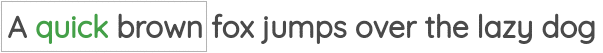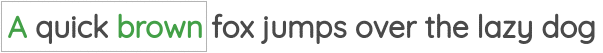### Word2Vec的局限

1、词汇表外（OOV）单词：在Word2Vec中，为每个单词创建一个嵌入。因此，它不能处理任何它在训练中没有遇到的单词。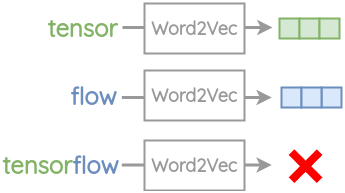2、单词形态：对于词根相同的单词，比如“eat”和“eaten”，Word2Vec不做任何参数共享。每一个单词都是根据它出现的上下文来学习的。因此，可以利用单词的内部结构来提高处理效率。### FastText的改进

1、子词生成：针对一个单词，我们获取长度3-6个字符的n-gram。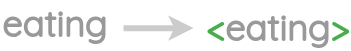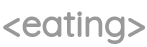Word Length(n) Character n-grams eating 3 eating 4 eating 5 eating 6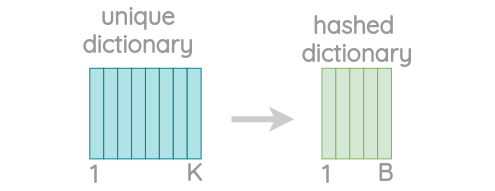2、包含负采样的Skip-gram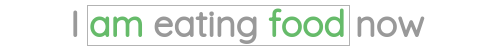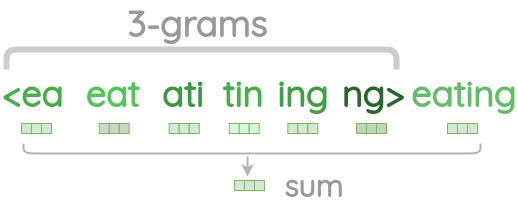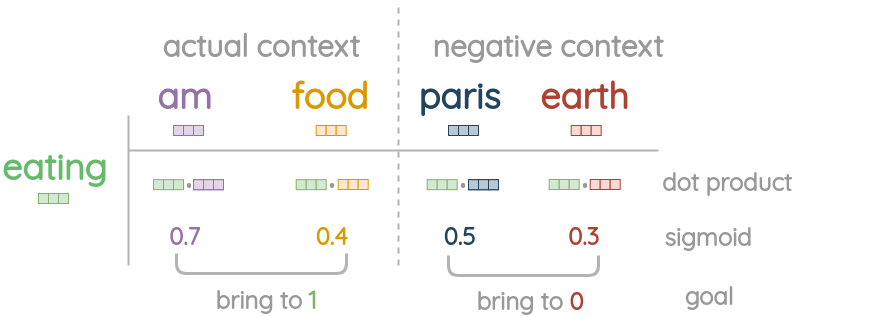### FastText总结

1、对于像捷克语和德语这样形态丰富的语言，FastText可以显著提高句法单词类比任务的性能。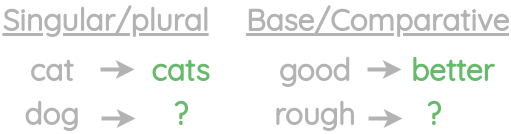word2vec-skipgram word2vec-cbow fasttext Czech 52.8 55.0 77.8 German 44.5 45.0 56.4 English 70.1 69.9 74.9 Italian 51.5 51.8 62.7

2、与Word2Vec相比，FastText在语义类比任务上的性能有所下降。word2vec-skipgram word2vec-cbow fasttext Czech 25.7 27.6 27.5 German 66.5 66.8 62.3 English 78.5 78.2 77.8 Italian 52.3 54.7 52.3

3、由于增加了n-grams的开销，FastText的训练速度比普通skipgram慢5倍

4、将子词信息与字符n-grams结合使用，在单词相似性任务中比CBOW和skip-gram基线具有更好的性能。用子词求和来表示单词外的单词比指定空向量具有更好的性能。

 skipgram cbow fasttext(null OOV) fasttext(char-ngrams for OOV) Arabic WS353 51 52 54 55 GUR350 61 62 64 70 German GUR65 78 78 81 81 ZG222 35 38 41 44 English RW 43 43 46 47 WS353 72 73 71 71 Spanish WS353 57 58 58 59 French RG65 70 69 75 75 Romanian WS353 48 52 51 54 Russian HJ 69 60 60 66

### FastText与Word2Vec

fastText的其中的一个作者是Thomas Mikolov。也正是这个人在谷歌的时候，带领团队在2012年提出了word2vec代替了one-hot编码，将词表示为一个低维连续嵌入，极大促进了NLP的发展。14年她去了脸书，然后提出了word2vec的改进版：fasttext。所以fastText和word2vec在结构上很相似。

• 图模型结构很像，都是采用 embedding 向量的形式，得到 word 的隐向量表达。
• 采用很多相似的优化方法，比如使用 Hierarchical softmax 优化训练和预测中的打分速度。
• 训练词向量时，两者都是无监督算法。输入层是 context window 内的 term。输出层对应的是每一个 term，计算某 term 的概率最大；
• 在使用层次softmax的时候，huffman 树叶子节点处是训练语料里所有词的向量。

• 模型的输出层：word2vec的输出层，对应的是每一个term，计算某term的概率最大；而fasttext的输出层对应的是分类的label。不过不管输出层对应的是什么内容，其对应的vector都不会被保留和使用；
• 模型的输入层：word2vec的输入层，是 context window 内的term；而fasttext 对应的整个sentence的内容，包括term，也包括 n-gram的内容；
• 两者本质的不同，体现在 Hierarchical softmax的使用。
• Wordvec的目的是得到词向量，该词向量 最终是在输入层得到，输出层对应的Hierarchical softmax也会生成一系列的向量，但最终都被抛弃，不会使用。
• fasttext则充分利用了Hierarchical softmax的分类功能，遍历分类树的所有叶节点，找到概率最大的label（一个或者N个）

FastText的优点：

• 适合大型数据+高效的训练速度：能够训练模型“在使用标准多核CPU的情况下10分钟内处理超过10亿个词汇”，特别是与深度模型对比，fastText能将训练时间由数天缩短到几秒钟。使用一个标准多核 CPU，得到了在10分钟内训练完超过10亿词汇量模型的结果。此外， FastText还能在五分钟内将50万个句子分成超过30万个类别。
• 支持多语言表达：利用其语言形态结构，FastText能够被设计用来支持包括英语、德语、西班牙语、法语以及捷克语等多种语言。它还使用了一种简单高效的纳入子字信息的方式，在用于像捷克语这样词态丰富的语言时，这种方式表现得非常好，这也证明了精心设计的字符 n-gram 特征是丰富词汇表征的重要来源。FastText的性能要比时下流行的word2vec工具明显好上不少，也比其他目前最先进的词态词汇表征要好。
• FastText专注于文本分类，在许多标准问题上实现当下最好的表现（例如文本倾向性分析或标签预测）。
• 比word2vec更考虑了相似性，比如FastText 的词嵌入学习能够考虑 english-born 和 british-born 之间有相同的后缀，但 word2vec 却不能。

## FastText实现细节

### 模型架构

FastText的架构和word2vec中的CBOW的架构类似，因为它们的作者Tomas Mikolov，而且确实FastText也算是word2vec所衍生出来的。

CBOW的架构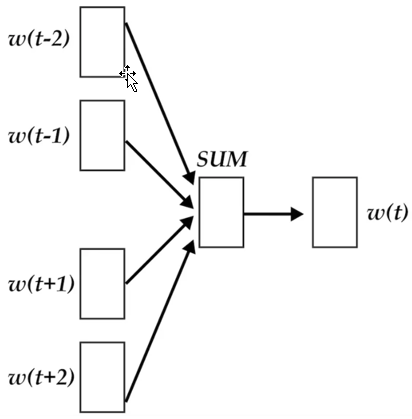fastText模型架构fastText与CBOW的不同点：

• CBOW的输入是目标单词的上下文，fastText的输入是多个单词及其n-gram特征，这些特征用来表示单个文档
• CBOW的输入单词被one-hot编码过，fastText的输入特征是被embedding过
• CBOW的输出是目标词汇，fastText的输出是文档对应的类标

### Hierarchical softmax

Softmax回归（Softmax Regression）又被称作多项逻辑回归（multinomial logistic regression），它是逻辑回归在处理多类别任务上的推广。

$$J(\theta)=-[\sum_{i=1}^{m} y^{(i)} \log h_{\theta}(x^{(i)})+(1-y^{(i)}) \log (1-h_{\theta}(x^{(i)}))]$$

$$h_{\theta}(x)=[\begin{array}{c}{P(y=1 | x ; \theta)} \\ {P(y=2 | x ; \theta)} \\ {\vdots} \\ {P(y=K | x ; \theta)}\end{array}]=\frac{1}{\sum_{j=1}^{K} e^{\theta(j)^{T} x}}[\begin{array}{c}{e^{\theta^{(1)} x} x} \\ {e^{\theta(2)^{T} x}} \\ {\vdots} \\ {e^{\theta^{(K)^{T}} x}}\end{array}]$$

$$J(\theta)=-[\sum_{i=1}^{m} \sum_{k=1}^{K} 1\{y^{(i)}=k\} \log \frac{e^{\theta^{(k) T} x^{(i)}}}{\sum_{j=1}^{K} e^{\theta(j) T_{x^{(i)}}}}]$$

\begin{aligned} J(\theta) &=-[\sum_{i=1}^{m} y^{(i)} \log h_{\theta}(x^{(i)})+(1-y^{(i)}) \log (1-h_{\theta}(x^{(i)}))] \\ &=-\sum_{i=1}^{m} \sum_{k=0}^{1} 1\{y^{(i)}=k\} \log P(y^{(i)}=k | x^{(i)} ; \theta) \\ &=-\sum_{i=1}^{m} \sum_{k=0}^{1} 1\{y^{(i)}=k\} \log \frac{e^{\theta^{(k) T} x^{(i)}}}{\sum_{j=1}^{K} e^{\theta(j) T_{x}(i)}} \end{aligned}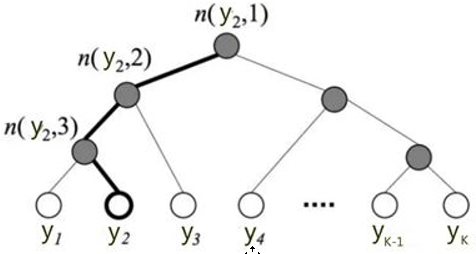$$\mathrm{P}(y_{j})=\prod_{i=1}^{L(y_{j})-1} \sigma(\left \| n(y_{j}, l+1)=L C(n(y_{j}, l))\right \| \cdot \theta_{n(y_{j}, l)}^{T} X)$$

\begin{aligned} \mathrm{P}(y_{2}) &=\mathrm{P}(n(y_{2}, 1), \text { left }) \cdot \mathrm{P}(n(y_{2}, 2), \text { left }) \cdot \mathrm{P}(n(y_{2}, 3), \text { right }) \\ &=\sigma(\theta_{n(y_{2}, 1)}^{T} X) \cdot \sigma(\theta_{n(y_{2}, 2)}^{T} X) \cdot \sigma(-\theta_{n(y_{2}, 3)}^{T} X) \end{aligned}

### N-gram

word2vec把语料库中的每个单词当成原子的，它会为每个单词生成一个向量。这忽略了单词内部的形态特征，比如：“book” 和“books”， “apple” 和“apples”，这两个例子中，两个单词都有较多公共字符，即它们的内部形态类似，但是在传统的word2vec中，这种单词内部形态信息因为它们被转换成不同的id丢失了。

“<ap”, “app”, “ppl”, “ple”, “le>”

• 对于低频词生成的词向量效果会更好。因为它们的n-gram可以和其它词共享。
• 对于训练词库之外的单词，仍然可以构建它们的词向量。我们可以叠加它们的字符级n-gram向量。

### FastText的优化

product quantization是一种保存数据间距离的压缩技术。PQ 用一个码本来近似数据，与传统的 keams 训练码本不同的是， PQ 将数据空间划分为 k 个子空间，并分别用 kmeans 学习子空间码本。数据的近似和重建均在子空间完成，最终拼接成结果。在 fasttext 中，子空间码本大小为 256，可以用 1 byte 表示。子空间的数量在 [2, d/2] 间取值。除了用 PQ 对数据进行量化压缩，fasttext 还提供了对分类系数的 PQ 量化选项。PQ 的优化能够在不影响分类其表现的情况下，将分类模型压缩为原大小的 $\frac{1}{10}$。

fasttext 提供了一个诱导式裁剪字典的算法，保证裁剪后的字典内容覆盖了所有的文章。具体而言，fasttext 存有一个保留字典，并在线处理文章，如果新的文章没有被保留字典涵盖，则从该文章中提取一个 norm 最大的词和其子串加入字典中。字典裁剪能够有效将模型的数量减少，甚至到原有的 $\frac{1}{100}$。

## FastText的使用

# -*- coding: utf-8 -*-
import jieba
import os
import fasttext

def get_data():
# 清华大学的新闻分类文本数据集下载：https://thunlp.oss-cn-qingdao.aliyuncs.com/THUCNews.zip
data_dir = 'D:\\迅雷下载\\THUCNews\\THUCNews\\财经'

with open("finance_news_cut.txt", "w", encoding='utf-8') as f:
for file_name in os.listdir(data_dir):
print(file_name)
file_path = data_dir + os.sep + file_name
with open(file_path, 'r', encoding='utf-8') as fr:
seg_text = jieba.cut(text.replace("\t", " ").replace("\n", " "))
outline = " ".join(seg_text)
f.write(outline)
f.flush()

def train_model():
model = fasttext.train_unsupervised('finance_news_cut.txt')
model.save_model("news_fasttext.model.bin")

def test_model():
print(model.words)
print(model.get_word_vector("股票"))
print(model.get_nearest_neighbors('股票'))

if __name__ == "__main__":
pass
# get_data()
# train_model()
test_model()


AttributeError: ‘_FastText’ object has no attribute ‘get_nearest_neighbors’

git clone https://github.com/facebookresearch/fastText.git
cd fastText
pip install .

# -*- coding: utf-8 -*-
import jieba
import os
import fasttext

def get_data():
# 清华大学的新闻分类文本数据集下载：https://thunlp.oss-cn-qingdao.aliyuncs.com/THUCNews.zip
data_dir = 'D:\\迅雷下载\\THUCNews\\THUCNews'

# 生成训练数据&测试数据
with open("news_fasttext_train.txt", "w", encoding='utf-8') as train_f, open("news_fasttext_test.txt", "w",
encoding='utf-8') as test_f:
for category in os.listdir(data_dir):
print(category)
category_path = data_dir + os.sep + category
count = 0
for file_name in os.listdir(category_path):
file_path = data_dir + os.sep + category + os.sep + file_name
with open(file_path, 'r', encoding='utf-8') as fr:
count += 1
seg_text = jieba.cut(text.replace("\t", " ").replace("\n", " "))
outline = " ".join(seg_text)
outline = outline + "\t__label__" + category + "\n"
print(count)
if count < 10000:
train_f.write(outline)
train_f.flush()
continue
elif count < 20000:
test_f.write(outline)
test_f.flush()
continue
else:
break

def train_model():
model = fasttext.train_supervised("news_fasttext_train.txt", label_prefix="__label__")
model.save_model("news_fasttext.model.bin")

def test_model():
result = model.test("news_fasttext_test.txt")
print('precision：', result)
# fasttext只提供全部结果的p值和r值，想要统计不同分类的结果，就需要自己写代码来实现了。
labels_right = []
texts = []
with open("news_fasttext_test.txt", encoding='utf-8') as fr:
for line in fr:
line = line.rstrip()
labels_right.append(line.split("\t").replace("__label__", ""))
texts.append(line.split("\t"))
labels_predict = [term for term in model.predict(texts)]  # 预测输出结果为二维形式
text_labels = list(set(labels_right))
text_predict_labels = list(set(labels_predict))

A = dict.fromkeys(text_labels, 0)  # 预测正确的各个类的数目
B = dict.fromkeys(text_labels, 0)  # 测试数据集中各个类的数目
C = dict.fromkeys(text_predict_labels, 0)  # 预测结果中各个类的数目
for i in range(0, len(labels_right)):
B[labels_right[i]] += 1
C[labels_predict[i]] += 1
if labels_right[i] == labels_predict[i].replace('__label__', ''):
A[labels_right[i]] += 1

# 计算准确率，召回率，F值
for key in B:
try:
r = float(A[key]) / float(B[key])
p = float(A[key]) / float(C['__label__' + key])
f = p * r * 2 / (p + r)
print("%s:\t p:%f\t r:%f\t f:%f" % (key, p, r, f))
except:
print("error:", key, "right:", A.get(key, 0), "real:", B.get(key, 0), "predict:", C.get(key, 0))

if __name__ == "__main__":
pass
get_data()
# train_model()
# test_model()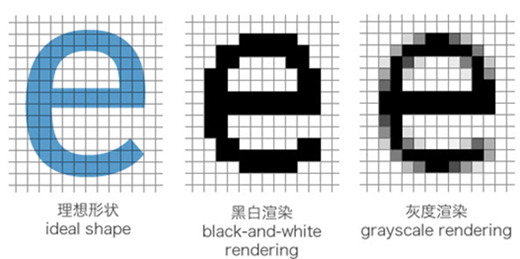##### 网站与APP开发中的字体设置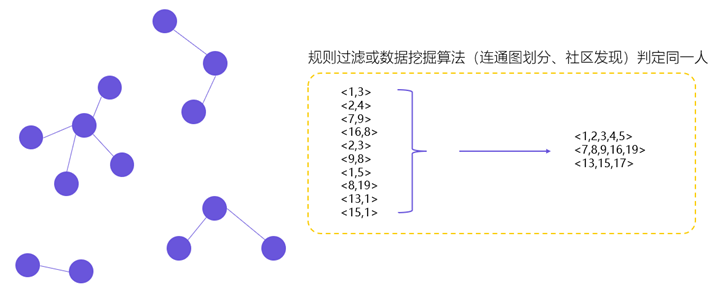##### 用户体系搭建之ID-Mapping